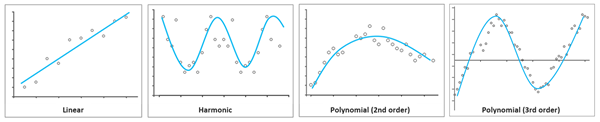# 生成趋势函数

## 备注

Mann-Kendall 和 Seasonal-Kendall 测试可用于确定数据中是否存在单调性趋势。上述测试为非参数测试，这意味着它们不会假设具体的数据分布。Mann-Kendall 测试不会考虑序列相关性或季节性影响。如果数据为季节性数据，则 Seasonal-Kendall 测试更为适合。

• 波段 1 = Sen 斜率
• 波段 2 = p 值
• 波段 3 = Mann-Kendall 得分 (S)
• 波段 4 = S 方差
• 波段 5 = Z 得分

Mann-Kendall 或 Seasonal-Kendall 测试的输出可用于确定多维时间序列中的哪些像素具有统计显著性趋势。您可以将此信息与线性、谐波或多项式趋势分析结合使用，以提取时间序列中的重要趋势。您可以生成包含像素（像素带有显著 p 值）的掩膜，将该掩膜应用于多维栅格，以及将此掩膜多维栅格用作工具的输入，以执行线性、谐波或多项式趋势分析。• 波段 1 = 斜率
• 波段 2 = 截距
• 波段 3 = 均方根误差 (RMSE) 或最佳拟合线周围的误差

• 波段 1 = Polynomial_2
• 波段 2 = Polynomial_1
• 波段 3 = Polynomial_0
• 波段 4 = RMSE

• 波段 1 = Polynomial_3
• 波段 2 = Polynomial_2
• 波段 3 = Polynomial_1
• 波段 4 = Polynomial_0
• 波段 5 = RMSE

• 波段 1 = 斜率
• 波段 2 = 截距
• 波段 3 = Harmonic_sin1
• 波段 4 = Harmonic_cos1
• 波段 5 = RMSE

• 波段 1 = 斜率
• 波段 2 = 截距
• 波段 3 = Harmonic_sin1
• 波段 4 = Harmonic_cos1
• 波段 5 = Harmonic_sin2
• 波段 6 = Harmonic_cos2
• 波段 7 = RMSE

## 参数

• 线性 - 拟合沿线性趋势线的变量的像素值。这是默认设置。
• 谐波 - 拟合沿谐波趋势线的变量的像素值。
• 多项式 - 拟合沿二阶多项式趋势线的变量的像素值。
• Mann-Kendall - 变量像素值将使用 Mann-Kendall 趋势测试进行评估。
• Seasonal Kendall - 变量像素值将使用 Seasonal-Kendall 趋势测试进行评估。

• - 谐波周期的长度单位为天。
• - 谐波周期的长度单位为年。这是默认设置。

• 选中 - 分析将包括沿给定维度的所有有效像素，并忽略所有 NoData 像素。这是默认设置。
• 未选中 - 如果沿给定维度的像素包含任意 NoData 值，则分析结果将变为 NoData。

RMSE

• 选中 - 该函数完成后，将计算 RMSE 并显示在详细信息中。这是默认设置。
• 未选中 - 将不计算 RMSE。

R 平方

• 选中 - 该函数完成后，将计算 R 平方并显示在详细信息中。
• 未选中 - 将不计算 R 平方。这是默认设置。

• 选中 - 该函数完成后，将计算 p 值并显示在详细信息中。
• 未选中 - 将不计算 p 值。这是默认设置。

• 天 - 季节性期间的长度单位为天。这是默认设置。
• 月 - 季节性期间的长度单位为月。

## 回归类型

• 线性 - 线性趋势线是最佳拟合直线，可用于估计简单的线性关系。线性趋势可突出显示以稳定速率增加或减少的变化率。线性趋势线的公式如下：
• y = 像素变量值。
• x = 维度值。
• ß0 = y 截距。
• ß1 = 线性斜率或变化率。

ß1 > 0 表示增加趋势。

ß1 < 0 表示减少趋势。

• 多项式 - 多项式趋势线是一条曲线，可用于波动的数据。在这种情况下，多项式的阶数值用于指示发生的最大波动数。多项式趋势线的公式如下：
• y = 像素变量值
• x = 维度值
• ß0, ß1, ß2, ß3, ..., ßn = 常系数
• 谐波 - 谐波趋势线是周期性重复的曲线，最适用于描述遵循周期性模式的数据，例如季节性温度变化。谐波趋势线的公式如下：
• y = 像素变量值
• t = 儒略日
• ß0 = y 截距
• ß1 = 变化率
• α, γ = 年际或年内变化的系数
• ω = i
• f = 谐波频率